# Multi Timeframe Indicators - page 446495

3 Like Break need MTF

Can someone make this 3 line break indicator into a multi time frame,

3_line_break.mq4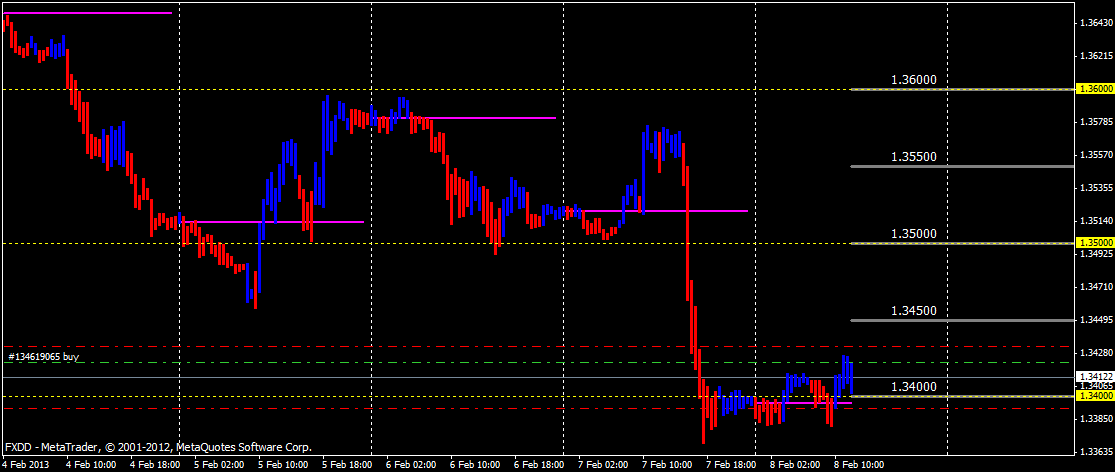Files:58

Dear

Thanks in advance . . .

Files:154516

AAREMM

You can check the one from this post : https://www.mql5.com/en/forum/173574/page238

AAREMM:
Dear

Thanks in advance . . .254

Dear toddanderson,

You can change the force of this indicator in the input tab. E.g. on 1 hour timeframe I use the 10 instead of the 3 which is the general input.

Try it and see the diffence.

Regards Marco58

Thank you . . . .again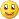31

Files:154516

You can not calculate multi time frame RSI the way that indicator calculates stochastic (stochastic too is an approximation, but a closer approximation than an RSI would be). See the comparison if one would calculate it the way how that stochastic is calculated : upper is a 1 hour stochastic and lower is what it would look like with that method applied at 15 minute chart. Marked with red lines only one example of different than it should be value, but all the values on a 15 minute chart are simply wrong and will deviate even more when the time frames differ more

1 hour chart :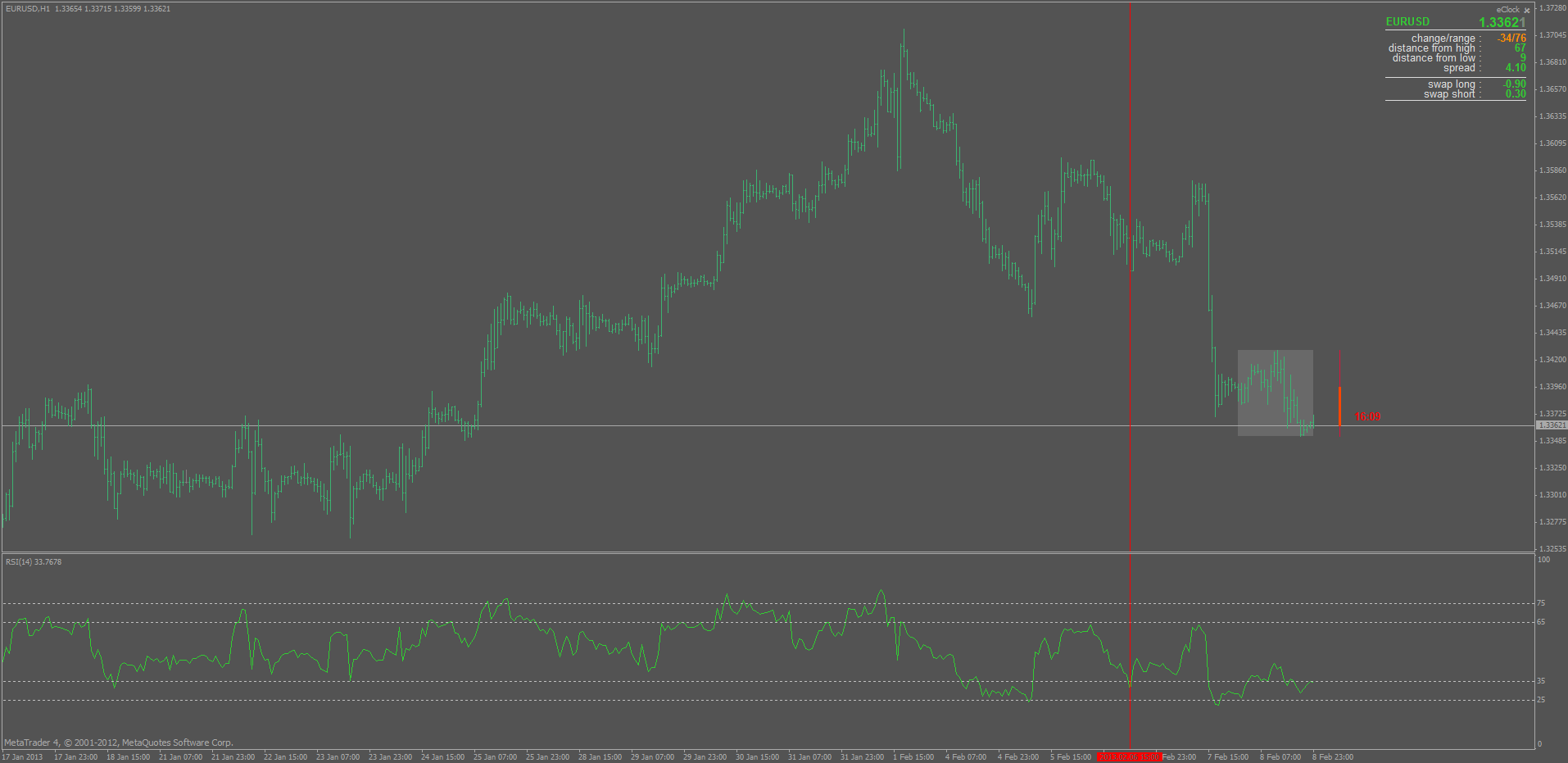15 minute chart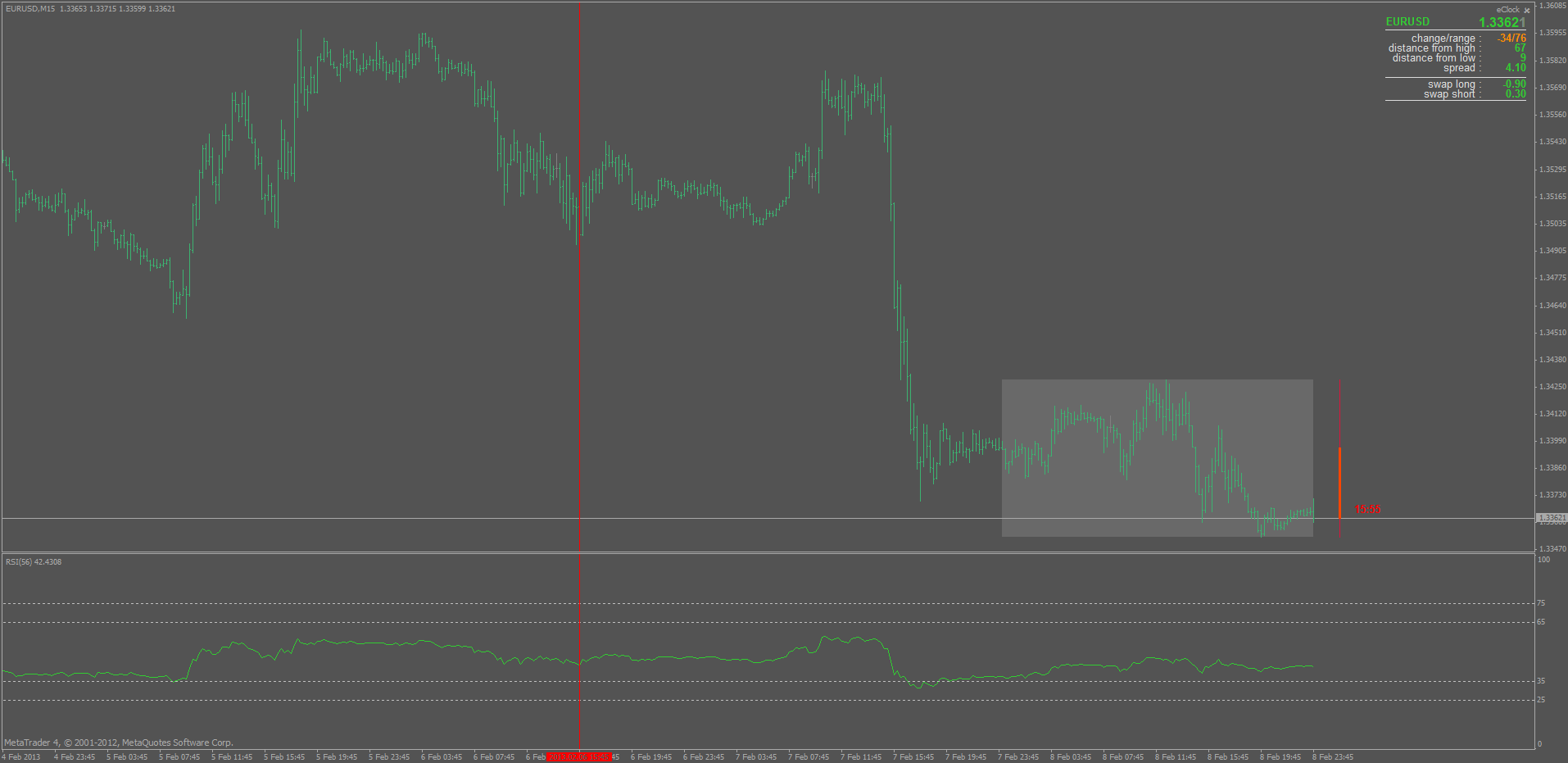Files:
rsi_15.gif 35 kb
rsi_60.gif 37 kb31

You can not calculate multi time frame RSI the way that indicator calculates stochastic (stochastic too is an approximation, but a closer approximation than an RSI would be). See the comparison if one would calculate it the way how that stochastic is calculated : upper is a 1 hour stochastic and lower is what it would look like with that method applied at 15 minute chart. Marked with red lines only one example of different than it should be value, but all the values on a 15 minute chart are simply wrong and will deviate even more when the time frames differ more

1 hour chart :15 minute chartHmmmm, ok.And what is your opinion indicator can be so constructed, that would be great MTF signals without jumping to the following algorithm, maybe WPR possible?154516

That way of calculating "other time frame" was used long time ago (some 40 years ago for example dr Alexander Elder used it in some cases) when people did not use computers for charting but were using paper and pen. Some can be approximated that way (like some moving averages, stochastic, ...) but those are few

As of WPR : set the stochastic slowing to 1, price to high/low, subtract 100 from it and you have a WPR: here is a comparison : upper is stochastic lower is WPR. Even Larry Williams (that claims that he invented WPR) uses stochastic indicator on some of his training videos (and calls it WPR)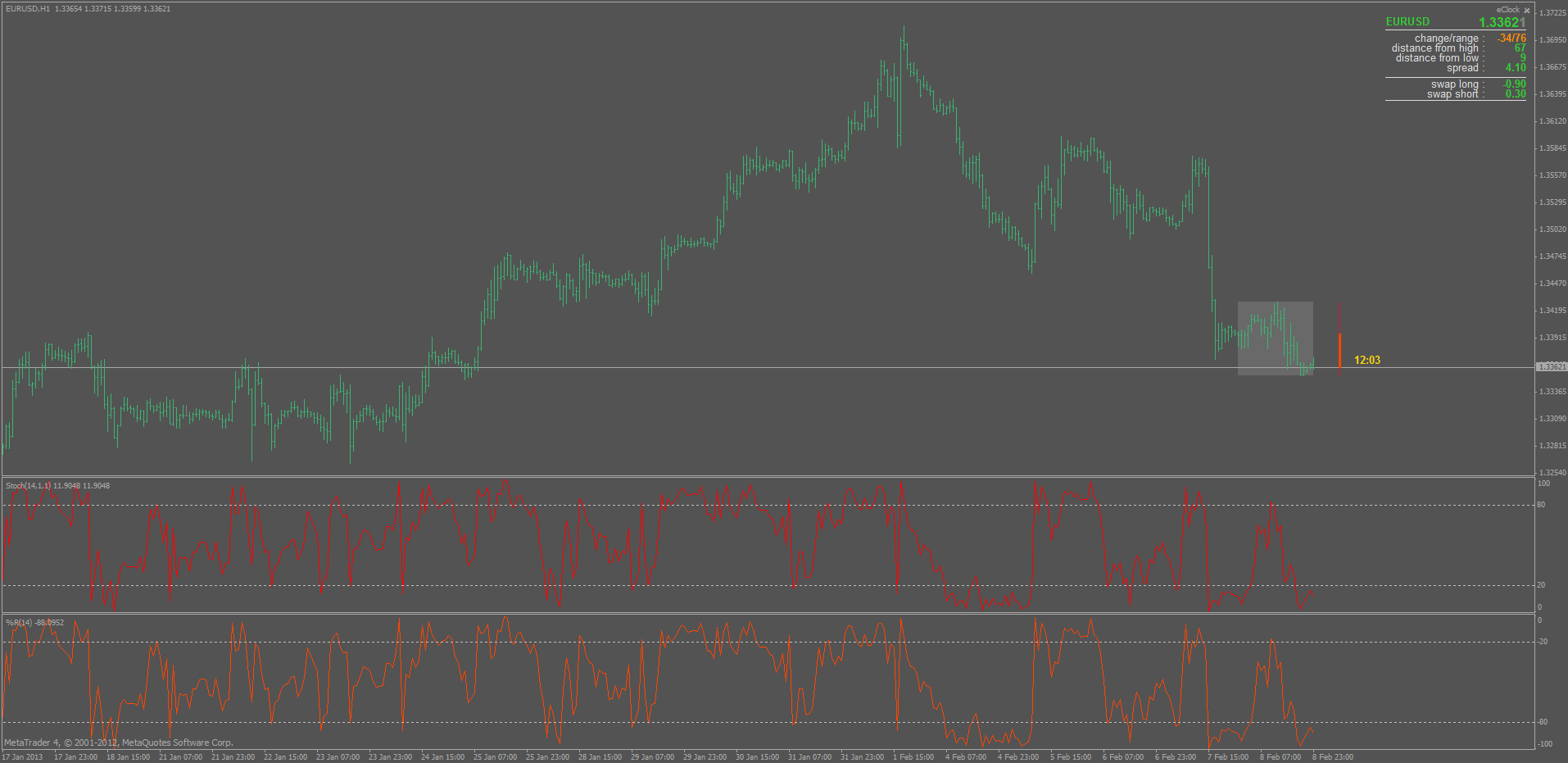Hmmmm, ok.And what is your opinion indicator can be so constructed, that would be great MTF signals without jumping to the following algorithm, maybe WPR possible?
Files:
sto_wpr.gif 46 kb31

As of WPR : set the stochastic slowing to 1, price to high/low, subtract 100 from it and you have a WPR: here is a comparison : upper is stochastic lower is WPR. Even Larry Williams (that claims that he invented WPR) uses stochastic indicator on some of his training videos (and calls it WPR)